# Calculate the current in all parts of this electric circuit

Karl Karlsson
Homework Statement:
In the circuit below, the output is 23 W across the resistor with the resistance 6 Ohm. Calculate the amount of current in all parts of the circuit as well as the polarity and EMF ε of the unknown battery.
Relevant Equations:
Basic formulas for electric circuits
In the circuit below, the output is 23 W across the resistor with the resistance 6 Ohm. Calculate the amount of current in all parts of the circuit as well as the polarity and EMF ε of the unknown battery.
Circuit: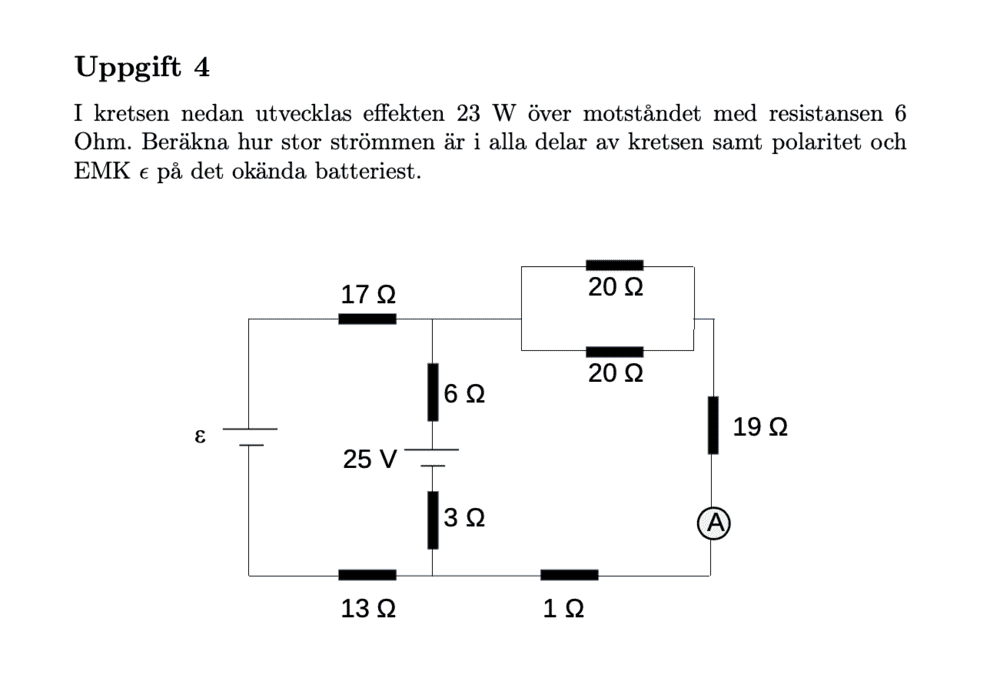My attempt: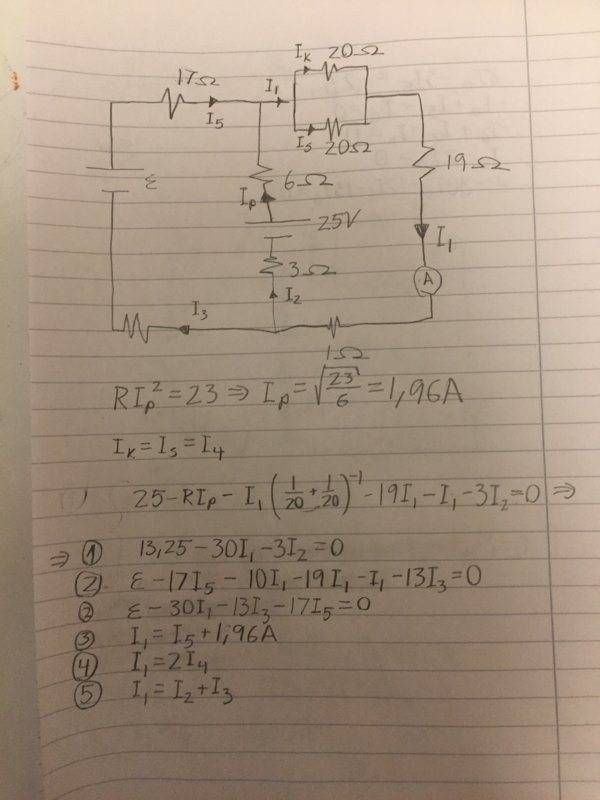I get 6 unknowns with 5 equations. I don't know how to find the last equation.

Thanks in beforehand!

Homework Helper
I don't understand your notation: what is ##I_K## ? Where is ##I_4## ?

Homework Helper
Ah, I see. And where is ##I_P## in the equations ? Only in the 13.25 ?
You make things difficult for yourself by not first simplifying the circuit. It can easily be reduced to three resistors and the two voltage sources.

•scottdave
Karl Karlsson
Ah, I see. And where is ##I_P## in the equations ? Only in the 13.25 ?
You make things difficult for yourself by not first simplifying the circuit. It can easily be reduced to three resistors and the two voltage sources.
Yes ##I_P## is only in 13.25, I wrote the numerical value directly instead of the symbol ##I_P##. I know I can reduce the ressistors to one equivalent ressistor in the part of the circuit where the current is ##I_0## but I already did that in equation 1. How do i reduce the circuit to an equivalent circuit with three resistors and the two voltage sources? How would you solve this? I know I can reduce the part where the current ##I_0## goes in the circuit but I did that in equation 1.

Homework Helper
Yes ##I_P ## is only in 13.25

What about the difference between ##I_P## and ##I_2## ? Would that be your missing eqn ?

How do i reduce the circuit to an equivalent circuit with three resistors
One in the left branch, one in the right branch and one in the middle branch!

Also makes you understand there are three relevant currents, not 8 or 9

•Merlin3189
Karl Karlsson
What about the difference between ##I_P## and ##I_2## ? Would that be your missing eqn ?
How can you calculate what ##I_P## - ##I_2## is?

One in the left branch, one in the right branch and one in the middle branch!

Also makes you understand there are three relevant currents, not 8 or 9
So you mean ##I_5## = ##I_3## and ##I_P## = ##I_2##? Can you show that please? And thanks for everything!

Homework Helper
2022 Award
These follow from the definition of an ideal battery: it provides zero resistance at fixed potential difference.

Homework Helper
current is charge flow. If the currents on the two ends of a battery would not be the same magnitude, the charge accumulation would quickly be enormous ! And with it the electrostatic energy. Compare with a water pump: what goes in must come out or the pump explodes (or implodes)

•hutchphd
Mentor
Bit of a nasty question. Just a casual perusal of the circuit let's one know that the constraint of 23 W dissipated by the 6 Ohm resistor could have current flowing in either direction. So there will be two solutions for the battery emf, one positive, one negative (for your chosen emf direction).

If we find the emf (or rather both emf's) then finding the rest of the currents should be rather straight forward.

Start by consolidating resistances other than the one 6 Ohm resistor in order to simplify the circuit: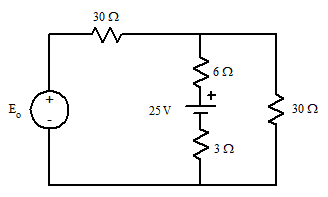Essentially the left branch and right branch resistors have been assimilated into single resistances.

We can see that the two 30 Ohm resistors form a voltage divider with E0. So plop that in and re-draw the circuit: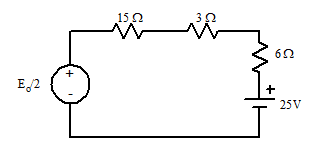From here you should be able to find the *two* values of Eo that will result in a 23 W dissipation by the 6 Ohm resistor. Once you have that, the rest follows from Kirchhoff.

Okay, I don't know if the Problem Originator intended for there to be multiple solutions to the problem, perhaps he/she intended only that the positive value for Eo be considered.

•scottdave
Homework Helper
We can see that the two 30 Ohm resistors form a voltage divider with E0. So plop that in and re-draw the circuit:
With respect, I find this dangerous and prefer a more straightforward approach, starting from the first layout. With the symbols and notation as in post #1: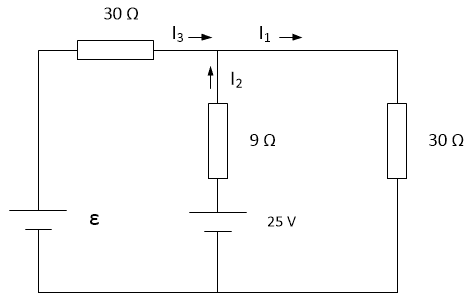I2 is known (except for the sign, as Karl -- and I-- misssed).

Postpone the ##\pm##; we have three unknowns: two currents and ##\varepsilon##.
And we have three equations: One simple Krichoff and two Ohm.

As for the sign: Two outcomes, one or both positive for ##\varepsilon##. the original circuit shows ##\varepsilon > 0## so maybe one can be written off.

Too lazy to do the math -- helper prerogative -- it's not my exercise anyway.
##\ ##

••scottdave, Merlin3189 and hutchphd
Mentor
With respect, I find this dangerous and prefer a more straightforward approach, starting from the first layout.
It's just a dash of Thevenin. Nothing to be wary of.

•scottdave
Homework Helper
Too old to change that for me. Perhaps something for Karl ... from whom we haven't heard for a while ...

Homework Helper
Gold Member
Ooops! Apologies to anyone who read my post. Just after I posted it, I looked at my working again and realized I'd made an arith error which changed my comment on magnitude of E and sign of I2.

Karl Karlsson
Thanks for all the great answers. I didn't know that the current had to be the same at both poles of the battery but as BvU mentioned that must be the case and it makes sense.

•BvU
Homework Helper
Thanks for all the great answers. I didn't know that the current had to be the same at both poles of the battery but as BvU mentioned that must be the case and it makes sense.
So what's your result?
Merlin deleted his first post, so the second hangs in the air.
I fumbled a little and found ##I_2<0## to get ##\varepsilon > 0##. Value of result satisfactory. : and in agreement with 2nd picture in #9: kudos for Thevenin and @gneill !

Last edited:
Karl Karlsson
So what's your result?
Merlin deleted his first post, so the second hangs in the air.
I fumbled a little and found ##I_2<0## to get ##\varepsilon > 0##. Value of result satisfactory.
I got ##I_3## = ##I_5## = -1,71A (according to my setup above) and the electromotive force was 44V with reversed polarity. ##I_p## = ##I_2## = 1.96A and ##I_1## = 0.25A. This agrees with the correct answers

•scottdave
Homework Helper
with reversed polarity.
Which doesn't agree with the picture. What do you get when you let ##I_2 = -1.96## A ?

Karl Karlsson
Which doesn't agree with the picture. What do you get when you let ##I_2 = -1.96## A ?
It does if you reverse the positive and negative pole of the battery with emf ε. In the answer sheet it said that the polarity of the battery was reversed

Homework Helper
Hmm, you happy, me a little less (bright idea about battery polarity not rewarded). Anyway: exercise solved !

•Karl Karlsson
Homework Helper
Gold Member
Bit of a nasty question. Just a casual perusal of the circuit let's one know that the constraint of 23 W dissipated by the 6 Ohm resistor could have current flowing in either direction. So there will be two solutions for the battery emf, one positive, one negative (for your chosen emf direction).

If we find the emf (or rather both emf's) then finding the rest of the currents should be rather straight forward.

Start by consolidating resistances other than the one 6 Ohm resistor in order to simplify the circuit:

View attachment 258126

Essentially the left branch and right branch resistors have been assimilated into single resistances.

We can see that the two 30 Ohm resistors form a voltage divider with E0. So plop that in and re-draw the circuit:

View attachment 258127

From here you should be able to find the *two* values of Eo that will result in a 23 W dissipation by the 6 Ohm resistor. Once you have that, the rest follows from Kirchhoff.

Okay, I don't know if the Problem Originator intended for there to be multiple solutions to the problem, perhaps he/she intended only that the positive value for Eo be considered.
I was wondering that too, except it asks for the polarity of the emf.

•BvU
Mentor
I was wondering that too, except it asks for the polarity of the emf.
Yup. So to be complete, you'd have to supply the two solution values with their polarities. Again, a bit of a nasty question for beginners.

•BvU
I completetly missed thatand navigated blindly on the visual impression from the diagram.•